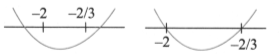Monotonicity
Question

# lf $\mathrm{f}\left(\mathrm{x}\right)={\mathrm{x}}^{3}+4{\mathrm{x}}^{2}+\mathrm{\lambda x}+1$is a monotonically decreasing function of x in the largest possible interval (-2, -2/3). Then

Moderate
Solution

## Here, ${\mathrm{f}}^{\mathrm{\prime }}\left(\mathrm{x}\right)\le 0$or    Then situations form f '(x) are as follows:Given that /(*) decreases in the largest possible interval $\left(-2,-\frac{2}{3}\right)$.Then $\mathrm{f}\text{'}\left(\mathrm{x}\right)=0$must have roots -2 and -2/3.Thus, product roots

Get Instant Solutions